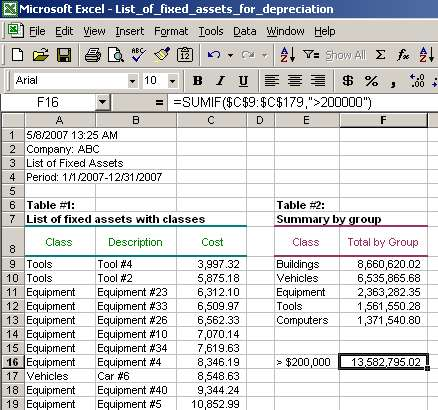# Sumif Function, Sumif Syntax, Sumif Examples

Finally, we copy the formula to cells F10-F13. Select cell F9, use key combination Ctrl+C to copy the formula, then select cells F10-F13 and use key combination Ctrl+V to paste the formula:The result is what we wanted - the list of fixed assets classes with their respective costs.

### 3) Sumif example #2: Summarize cost of fixed assets over a certain limit using SUMIF

Sometimes we want to summarize costs over a certain amount. For example, using the data from the above example, we may want to know the total value of all fixed assets with costs of more than \$200,000. In this case, we can again use SUMIF function.

Recall that the criteria may be a number, expression or text. Expression is what we will use to accomplish the task in this example:

=SUMIF ( \$C\$9:\$C\$179, ">200000" ), where

• \$C\$9:\$C\$179 is range
• ">200000" is criteria.

The third argument, sum_range, is omitted from the formula. That is because if sum_range is omitted, the cells in range are evaluated against the criteria and summed. So, cells C9-C179 serve as the range and the sum_range at the same time in the above formula:The result of the formula shows that the total of all fixed assets with individual costs of more than \$200,000 is 13,582,795.02.

You can also use less than ("<") if you need to summarize fixed assets under a certain amount.

Page 3 of 3

Back